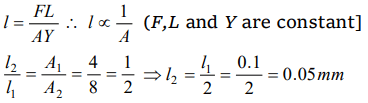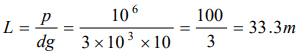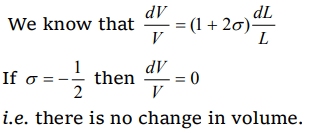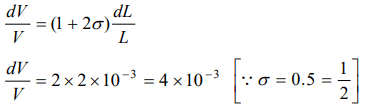## Mechanical Properties of Solids Questions and Answers Part-12

1.Young’s modulus of perfectly rigid body material is
a) Zero
b) Infinity
c) $1\times 10^{10}N\diagup m^{2}$
d) $10\times 10^{10}N\diagup m^{2}$

Explanation: Infinity

2. A wire of cross section $4 mm^{2}$ is stretched by 0.1mm by a certain weight. How far (length) will be wire of same material and length but of area $8 mm^{2}$ stretch under the action of same force
a) 0.05 mm
b) 0.10 mm
c) 0.15 mm
d) 0.20 mm

Explanation:3. A substance breaks down by a stress of 106 N/m2. If the density of the material of the wire is $3\times 10^{3}kg\diagup m^{3}$  , then the length of the wire of the substance which will break under its own weight when suspended vertically, is
a) 66.6 m
b) 60.0 m
c) 33.3 m
d) 30.0 m

Explanation:4. A rubber cord 10 m long is suspended vertically. How much does it stretch under its own weight (Density of rubber is $1500 kg\diagup m^{3}$ , $Y=5\times 10^{8}N\diagup m^{2}$   , $g= 10m\diagup s^{2}$  )
a) $15\times 10^{-4}m$
b) $7.5\times 10^{-4}m$
c) $12\times 10^{-4}m$
d) $25\times 10^{-4}m$

Explanation:5. The value of Poisson's ratio lies between
a) $-1 to \frac{1}{2}$
b) $-\frac{3}{4} to -\frac{1}{2}$
c) $-\frac{1}{2} to 1$
d) 1 to 2

Explanation:6. The Poisson's ratio cannot have the value
a) 0.7
b) 0.2
c) 0.1
d) 0.5

Explanation: Value of Poisson’s ratio lie in range of -1 to $\frac{1}{2}$

7. There is no change in the volume of a wire due to change in its length on stretching. The Poisson's ratio of the material of the wire is
a) + 0.50
b) – 0.50
c) 0.25
d) - 0.25

Explanation:8. A material has Poisson's ratio 0.50. If a uniform rod of it suffers a longitudinal strain of $2\times 10^{-3}$ , then the percentage change in volume is
a) 0.6
b) 0.4
c) 0.2
d) Zero

Explanation:Percentage change in volume = 4 * 10-1 = 0.4%

9. Four identical rods are stretched by same force. Maximum extension is produced in
a) $L =10cm, D =1mm$
b) $L =100cm, D =2mm$
c) $L =200cm, D =3mm$
d) $L =300cm, D =4mm$